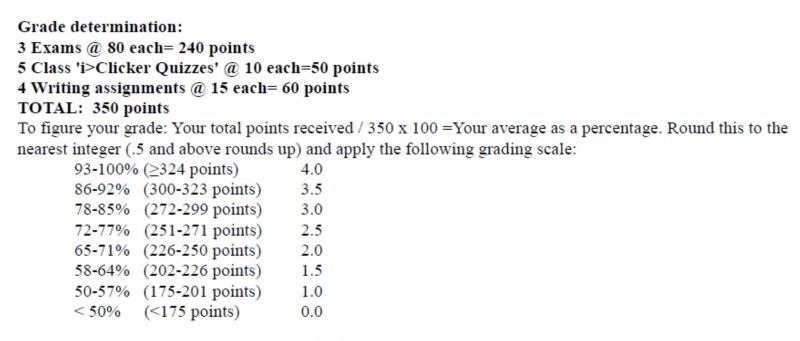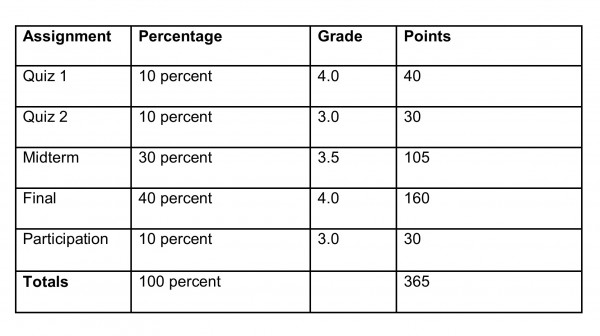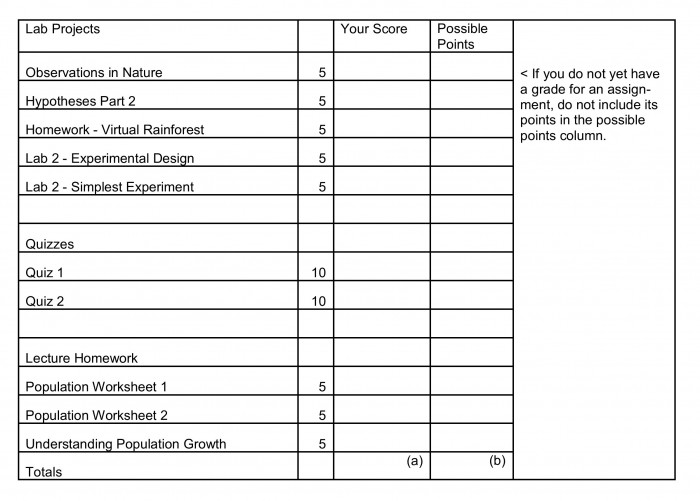As an Undergraduate Student it is important to know how to calculate your GPA, which will be reported to Potential Employers and Graduate schools you apply to. The information below will help you calculate your GPA thus far. You can also use it to calculate your anticipated GPA for the current semester.

To calculate GPA, use the following steps:
1. List each course you took or plan on taking and how many credits it is worth.
2. For each course, list the earned or expected grade.
3. Multiply the number of credits by the grade to get the “Grade Points” for each course.
5. Divide the Total Grade Points by the total number of Credit Hours attempted. Credit hours = the total number of credits you earned and attempted. (Note that you do not earn credits for courses with a grade of 0.0 at MSU)

EXAMPLE – You are interested in knowing your GPA and these are the grades you have thus far in your classes.57 Grade Points ÷ 15 Credits = 3.8 GPA

_________________________________

### HOW TO CALCULATE GRADES FOR EACH CLASS:

First and foremost, you should look at your syllabus for EACH class. The ways to calculate your grades depend on the method of your professor. But most (if not all) professors will include how they figure out points/percentages that determine grades for each student on the syllabus. Also check D2L for running totals that might be provided by your professor.

SAMPLE SYLLABUS

Your syllabus will include something like this “grade determination” chart. (NOTE: You should keep track of your grades and then you can plug them in to get an approximation for how you are doing.)1. List the assignments and allotted percentages that each is worth.
3. Multiply the amount that this assignment is worth by the grade to get the points for each assignment.
5. Divide the Total Grade Points by 100. This should give you an idea of your expected grade.

5. Divide the Grade Points by the number of assignments completed.365 points ÷ 100 = 3.65 Grade
70 points (for the quizzes) ÷ 20 (quiz percent) = 3.5 Grade (for the quizzes)

MSU grades are reported in increments of 0.5 (e.g. 3.0; 3.5; 4.0). Instructors may choose to give you an intermediate grade on an assignment. For example, you could earn a 3.3 on your midterm and 2.9 for participation. In addition, if you earn a 3.25 grade or similar intermediate grade in the class, your instructor may choose to round up or down to the nearest reportable grade for your final course grade.

Sample calculation of Lab:Your current percentage is a/b: __________(a)/__________(b) = ______________%

Are you satisfied with this grade?